Curvature of a triangle mesh, definition and computation.Defining and giving the formula to compute the curvature over a triangle mesh at some vertices.

Normal curvature

By intersecting a surface with a plane we see that the section defines a curve: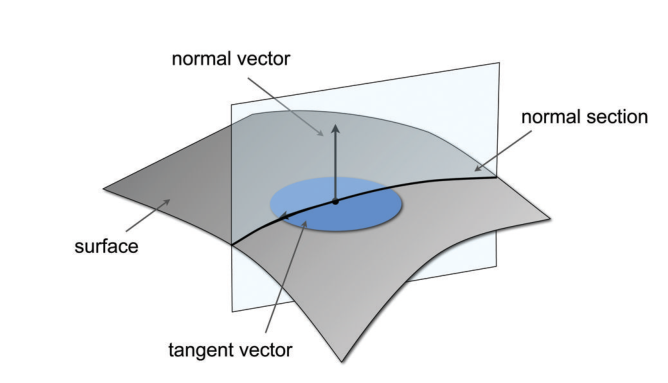The curvature of this curve is called 'normal curvature' since the plane is spanned through the normal $$\boldsymbol n$$ and an arbitrary tangent vector $$\boldsymbol t$$. Now, given a point $$\boldsymbol p$$ on the surface you can find an infinity of curvatures given the direction you choose for your tangent vector $$\boldsymbol t$$.

Principal curvatures

But how can we characterize the curvature of a surface at a given point when we seem to have an infinity of curvatures? Don't worry! It can be shown that when rotating the normal plane around $$\boldsymbol n$$ you can always find two 'principal curvatures' $$k_1$$ and $$k_2$$ respectively defining the maximal and minimal curvature at a given point $$\boldsymbol p$$ of the surface: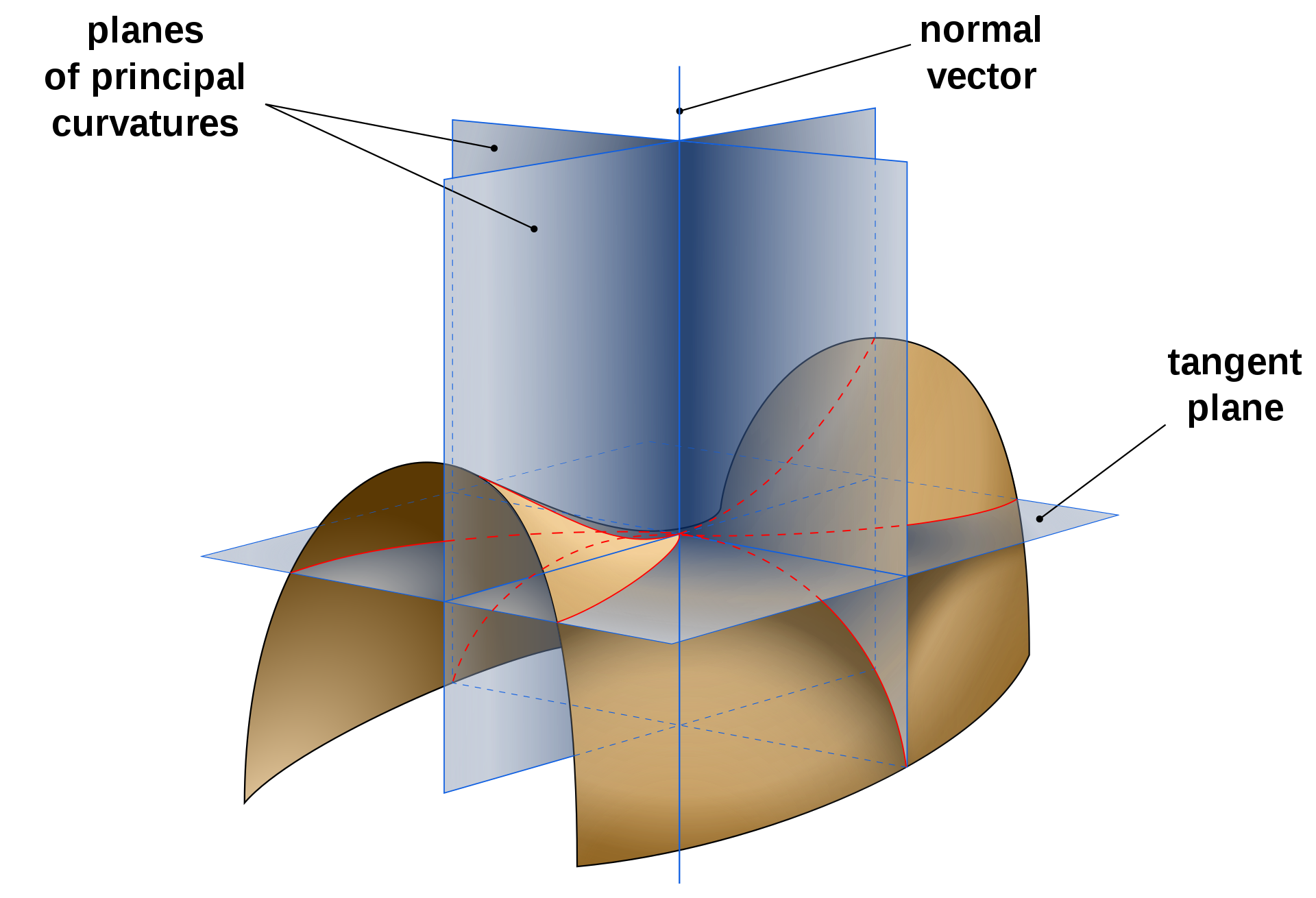In addition, the principal curvatures $$k_1$$ and $$k_2$$ are associated to the 'principal directions' $$\boldsymbol t_1$$ and $$\boldsymbol t_2$$ which are always found to be orthogonal to each other! What's more, you can define any 'normal curvature' $$k_n$$ as a combination of the principal curvatures $$k_1$$ and $$k_2$$ using Euler's theorem below:

$k_n = k_1\cos^2\theta + k_2\sin^2\theta$

Where $$\theta$$ is the angle between $$\boldsymbol t_1$$ (maximal curvature direction) and $$\boldsymbol t_n$$ the normal curvature.

Calculation

One can compute the principal curvatures $$k_1$$ and $$k_2$$ according to the Mean curvature $$H$$ and the Gaussian curvature $$k_g$$, don't worry we will define both $$H$$ and $$k_g$$ in the next sections:

\begin{align*} k_1 & = H + \sqrt{ H^2 - k_g} \\ k_2 & = H - \sqrt{ H^2 - k_g} \end{align*}

Gaussian curvature

The Gaussian is the product of principal curvatures $$k_g = k_1 * k_2$$ on a triangular mesh it can be computed at vertex $$\boldsymbol p_i$$ as follows:

$k_g = \frac{ 2 \pi - \sum \theta_j } { A_i }$

Where the angle $$\theta_j$$ is defined as: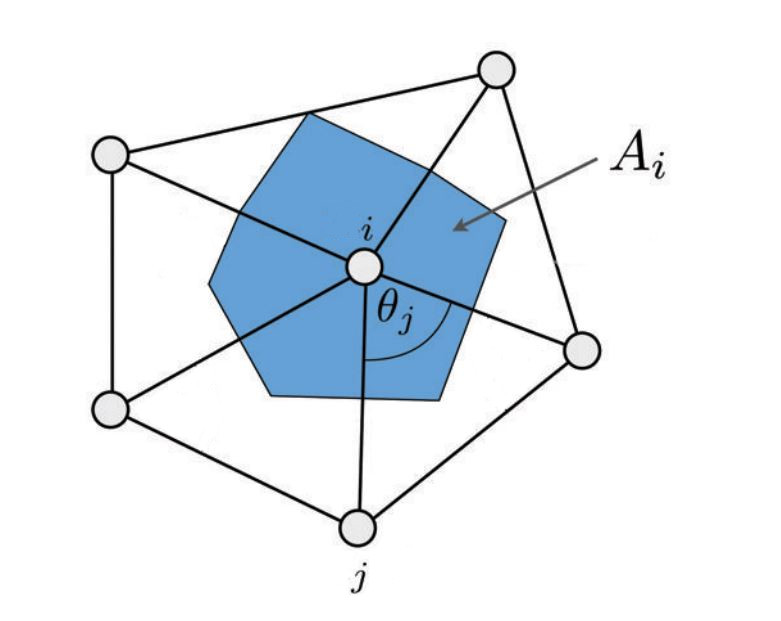$$A_i$$ can be computed as:

• 'mixed voronoi area'.
• 'barycentric cell area' (sum of the triangles' area adjacent to $$i$$ times 3): $$A_i = 3 \sum\limits_{{T_j \in \mathcal N(i)}} {area(T_j)}$$

Interpreting the Gaussian curvature's value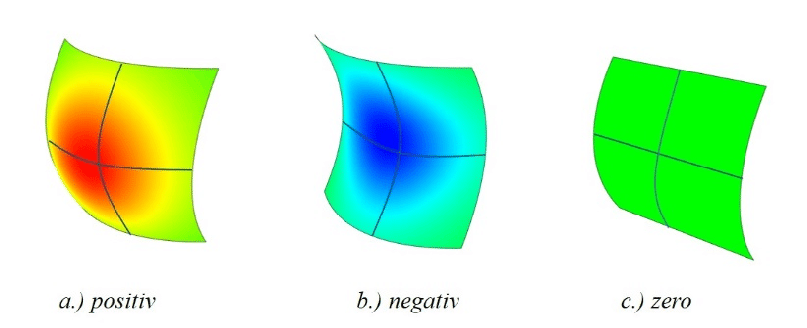It can help to locally characterize the shape:

• $$k_g > 0$$ means surface is locally a bowl-like shape. (elliptical point)
• $$k_g < 0$$ means surface is locally a saddle-like shape. (hyperbolic point)
• $$k_g = 0$$ means surface is locally flat in at least one direction (parabolic point)

Mean curvature

The mean curvature is the average of the principal curvatures:

$$H= \frac { k_1 + k_2 } { 2 }$$

In addition, the mean curvature can also be expressed using the Laplace operator:

$$H = \frac{ \| \Delta p_i \|}{ 2 }$$

In other words, to find the mean curvature for a triangle mesh, we need to apply the discrete Laplace operator at some vertex $$\boldsymbol p_i$$ as follows:

$$\Delta \vec p_i = \frac{1} {2A_i} \sum\limits_{{j \in \mathcal N(i)}} { (\cot \alpha_{ij} + \cot \beta_{ij}) (p_j - p_i) }$$

• $${j \in \mathcal N(i)}$$ is the list of vertices directly adjacent to the vertex $$i$$
• $$\cot \alpha_{ij} + \cot \beta_{ij}$$ are the famous cotangent weights (a real value)
• $$p_i, p_j, ...$$ vertices position.
• $$A_i$$ is the cell area as described earlier (a real value).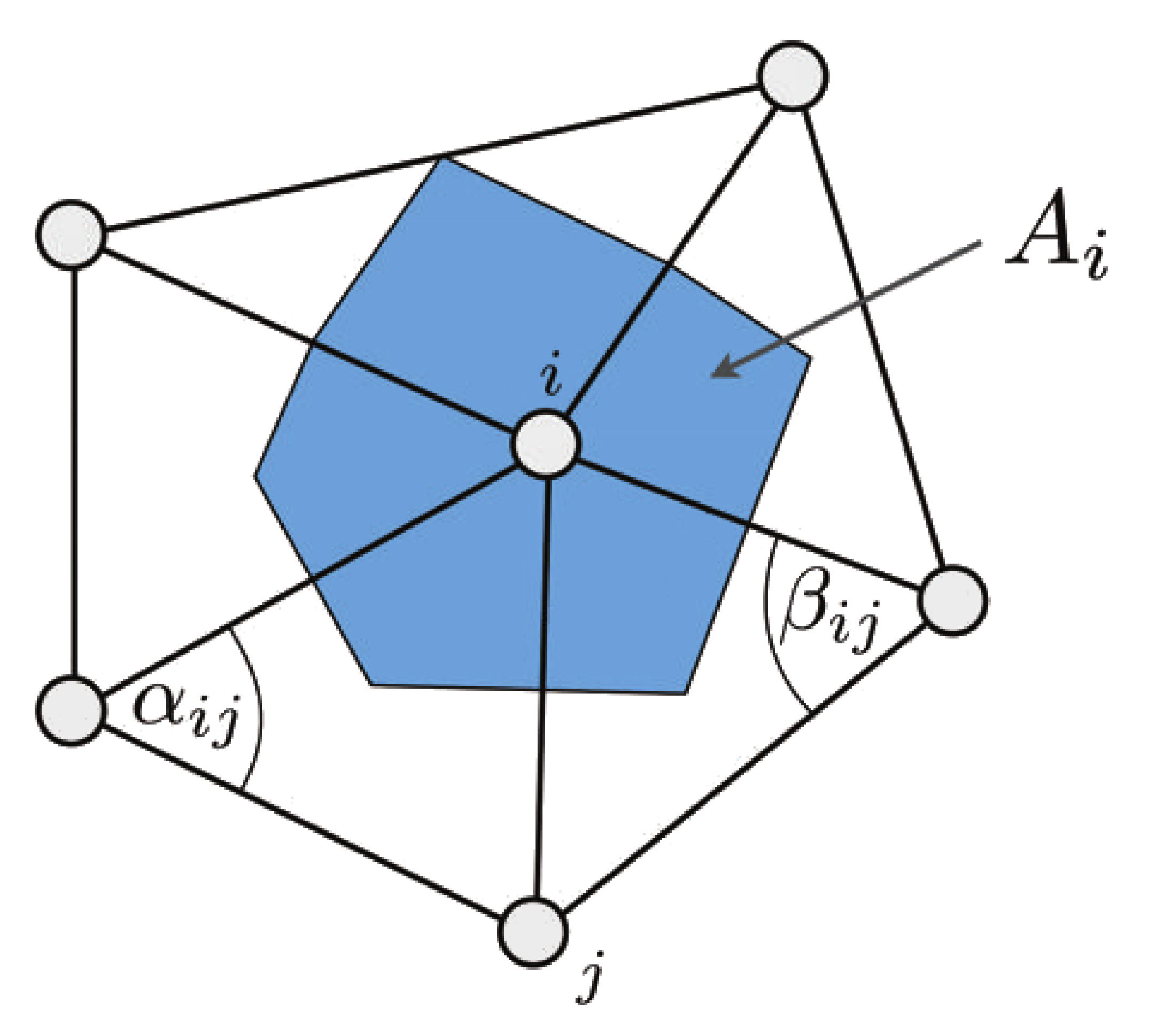Interpreting the Mean curvature's value

It does not characterize the shape but gives the amount of curvature in one way or another:

• $$H = 0$$ -> saddle or flat surface
• $$H > 0$$ -> amount of convexity (peak)
• $$H < 0$$ -> amount of concavity (pit)

Gaussian VS Mean curvature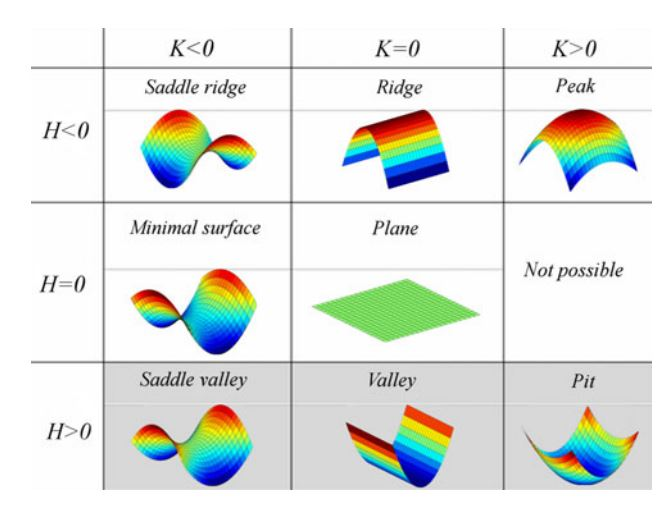References